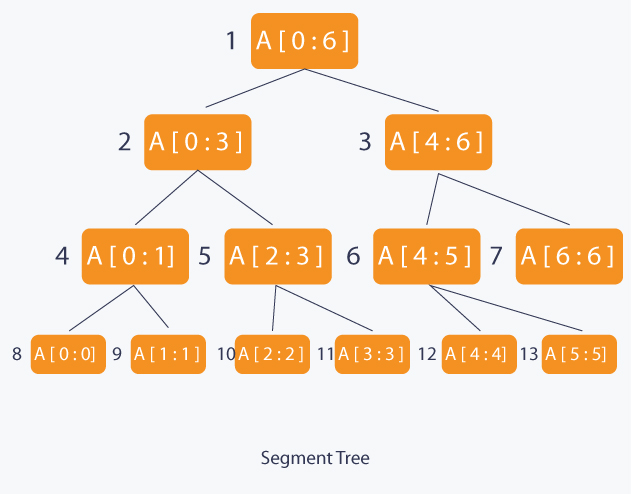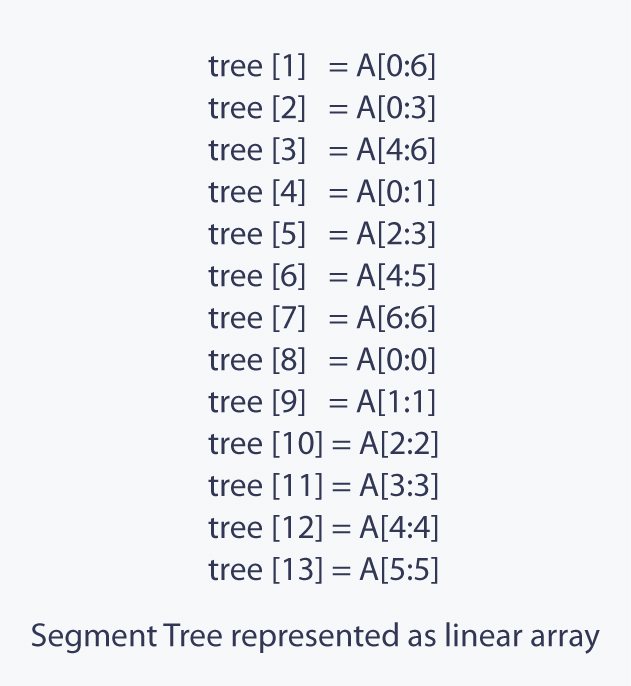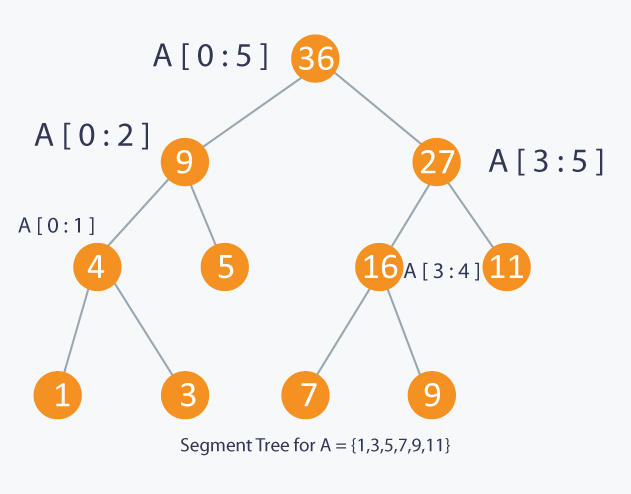Data Structures
Topics:
Segment Trees
• Arrays
• Stacks
• Queues
• Hash Tables
• Trees
• Disjoint Data Structures

# Segment Trees

Segment Tree is used in cases where there are multiple range queries on array and modifications of elements of the same array. For example, finding the sum of all the elements in an array from indices $L$ to $R$, or finding the minimum (famously known as Range Minumum Query problem) of all the elements in an array from indices $L$ to $R$. These problems can be easily solved with one of the most versatile data structures, Segment Tree.

# What is Segment Tree ?

Segment Tree is a basically a binary tree used for storing the intervals or segments. Each node in the Segment Tree represents an interval. Consider an array $A$ of size $N$ and a corresponding Segment Tree $T$:

1. The root of $T$ will represent the whole array $A[0:N-1]$.
2. Each leaf in the Segment Tree $T$ will represent a single element $A[i]$ such that $0 \le i \lt N$.
3. The internal nodes in the Segment Tree $T$ represents the union of elementary intervals $A[i:j]$ where $0 \le i \lt j \lt N$.

The root of the Segment Tree represents the whole array $A[0:N-1]$. Then it is broken down into two half intervals or segments and the two children of the root in turn represent the $A[0:(N-1) / 2]$ and $A[ (N-1) / 2 + 1 : (N-1) ]$. So in each step, the segment is divided into half and the two children represent those two halves. So the height of the segment tree will be $log_2 N$. There are $N$ leaves representing the $N$ elements of the array. The number of internal nodes is $N-1$. So, a total number of nodes are $2 \times N - 1$.

Once the Segment Tree is built, its structure cannot be changed. We can update the values of nodes but we cannot change its structure. Segment tree provides two operations:

1. Update: To update the element of the array $A$ and reflect the corresponding change in the Segment tree.
2. Query: In this operation we can query on an interval or segment and return the answer to the problem (say minimum/maximum/summation in the particular segment).

Implementation:

Since a Segment Tree is a binary tree, a simple linear array can be used to represent the Segment Tree. Before building the Segment Tree, one must figure what needs to be stored in the Segment Tree's node?.
For example, if the question is to find the sum of all the elements in an array from indices $L$ to $R$, then at each node (except leaf nodes) the sum of its children nodes is stored.

A Segment Tree can be built using recursion (bottom-up approach ). Start with the leaves and go up to the root and update the corresponding changes in the nodes that are in the path from leaves to root. Leaves represent a single element. In each step, the data of two children nodes are used to form an internal parent node. Each internal node will represent a union of its children’s intervals. Merging may be different for different questions. So, recursion will end up at the root node which will represent the whole array.

For $update()$, search the leaf that contains the element to update. This can be done by going to either on the left child or the right child depending on the interval which contains the element. Once the leaf is found, it is updated and again use the bottom-up approach to update the corresponding change in the path from that leaf to the root.

To make a $query()$ on the Segment Tree, select a range from $L$ to $R$ (which is usually given in the question). Recurse on the tree starting from the root and check if the interval represented by the node is completely in the range from $L$ to $R$. If the interval represented by a node is completely in the range from $L$ to $R$, return that node’s value.

The Segment Tree of array $A$ of size $7$ will look like :Take an example. Given an array $A$ of size $N$ and some queries. There are two types of queries:

1. Update: Given $idx$ and $val$, update array element $A[idx]$ as $A[idx] = A[idx] + val$.
2. Query: Given $l$ and $r$ return the value of $A[l] + A[l+1] + A[l+2] + ….. + A[r-1] + A[r]$ such that $0 \le l \le r \lt N$

Queries and Updates can be in any order.

Naive Algorithm:
This is the most basic approach. For every query, run a loop from $l$ to $r$ and calculate the sum of all the elements. So each query will take $O(N)$ time.

$A[idx] += val$ will update the value of the element. Each update will take $O(1)$.

This algorithm is good if the number of queries are very low compared to updates in the array.

Using Segment Tree:
First, figure what needs to be stored in the Segment Tree's node. The question asks for summation in the interval from $l$ to $r$, so in each node, sum of all the elements in that interval represented by the node. Next, build the Segment Tree. The implementation with comments below explains the building process.

void build(int node, int start, int end)
{
if(start == end)
{
// Leaf node will have a single element
tree[node] = A[start];
}
else
{
int mid = (start + end) / 2;
// Recurse on the left child
build(2*node, start, mid);
// Recurse on the right child
build(2*node+1, mid+1, end);
// Internal node will have the sum of both of its children
tree[node] = tree[2*node] + tree[2*node+1];
}
}


As shown in the code above, start from the root and recurse on the left and the right child until a leaf node is reached. From the leaves, go back to the root and update all the nodes in the path. $node$ represents the current node that is being processed. Since Segment Tree is a binary tree. $2 \times node$ will represent the left node and $2 \times node + 1$ will represent the right node. $start$ and $end$ represents the interval represented by the node. Complexity of $build()$ is $O(N)$.To update an element, look at the interval in which the element is present and recurse accordingly on the left or the right child.

void update(int node, int start, int end, int idx, int val)
{
if(start == end)
{
// Leaf node
A[idx] += val;
tree[node] += val;
}
else
{
int mid = (start + end) / 2;
if(start <= idx and idx <= mid)
{
// If idx is in the left child, recurse on the left child
update(2*node, start, mid, idx, val);
}
else
{
// if idx is in the right child, recurse on the right child
update(2*node+1, mid+1, end, idx, val);
}
// Internal node will have the sum of both of its children
tree[node] = tree[2*node] + tree[2*node+1];
}
}


Complexity of update will be $O(logN)$.

To query on a given range, check 3 conditions.

1. Range represented by a node is completely inside the given range
2. Range represented by a node is completely outside the given range
3. Range represented by a node is partially inside and partially outside the given range

If the range represented by a node is completely outside the given range, simply return 0. If the range represented by a node is completely within the given range, return the value of the node which is the sum of all the elements in the range represented by the node. And if the range represented by a node is partially inside and partially outside the given range, return sum of the left child and the right child. Complexity of query will be $O(logN)$.

int query(int node, int start, int end, int l, int r)
{
if(r < start or end < l)
{
// range represented by a node is completely outside the given range
return 0;
}
if(l <= start and end <= r)
{
// range represented by a node is completely inside the given range
return tree[node];
}
// range represented by a node is partially inside and partially outside the given range
int mid = (start + end) / 2;
int p1 = query(2*node, start, mid, l, r);
int p2 = query(2*node+1, mid+1, end, l, r);
return (p1 + p2);
}

Contributed by: Akash Sharma

?